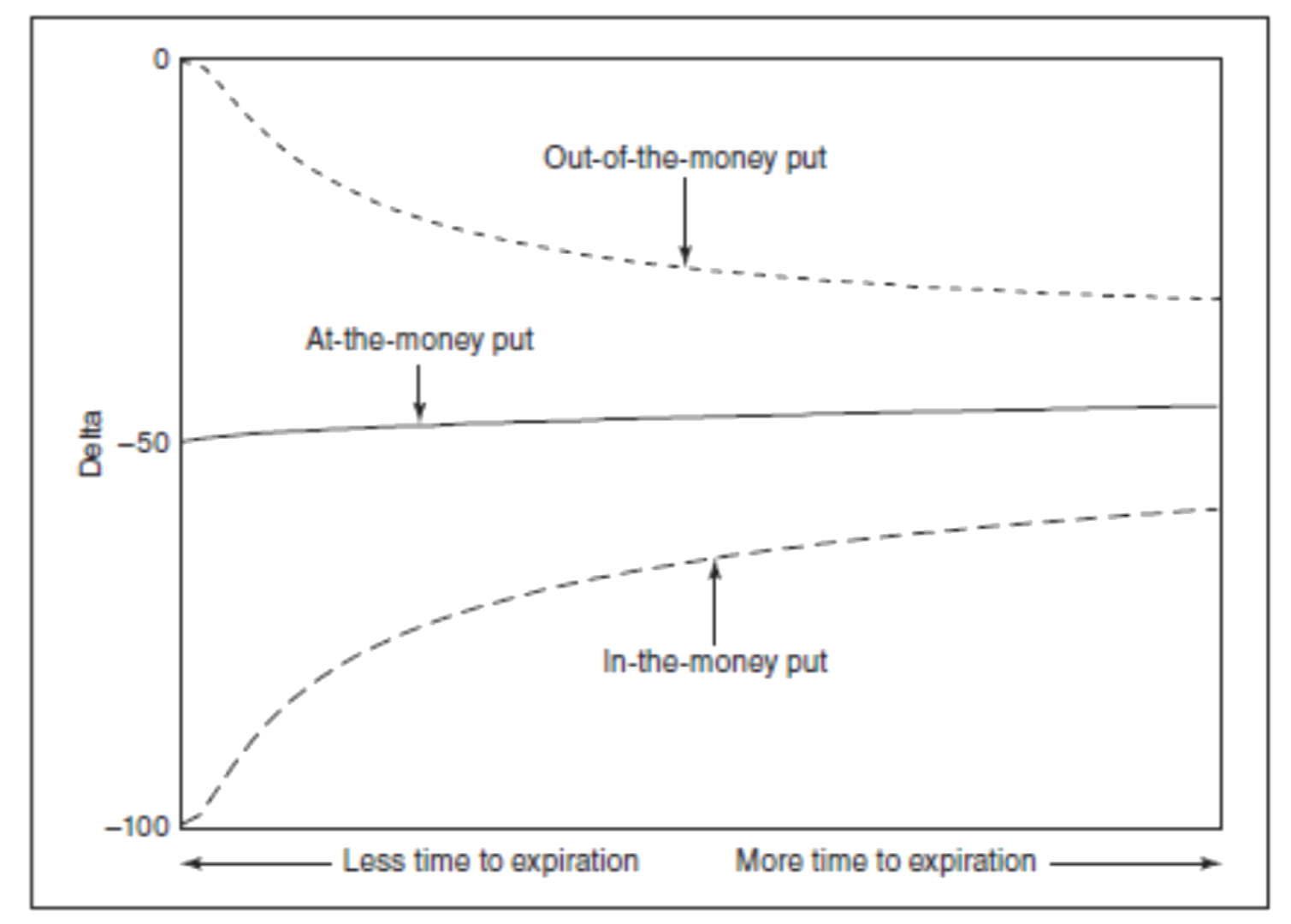CharmCharm

# Charm

In options trading, the second order option Greek, "charm" (also known as "delta decay") is a measurement of the change in an option's delta due to a change in time to expiration. It is a second-order derivative of the option price with respect to time. The interpretation of charm is that it tells us, how much the delta will change for a one -day decrease in time to expiration.

For example, if charm of a call option is 0.05, it means the delta of the option will increase by 0.05, if there is one-day decrease in time to expiration.

Charm =d(Delta)/ d(Time)

where delta is the option delta and time is the time to expiry.

Charm is an important option Greek because it can help traders manage their delta risk. If a trader has a long call position, for example, the charm is positive, then the delta of the option increases as time passes. This means that the trader may want to hedge their position by selling some of the underlying asset, to offset the increase in delta, in order to maintain a constant portfolio delta.

A negative Charm value means that the delta of the option will decrease as time to expiration decreases. As the time to expiration or days to expiry (DTE) decreases, the option becomes less valuable and more likely to expire out of the money. The Charm is used to measure this rate of change and helps traders manage the risk associated with the passage of time, as mentioned earlier.

Above is an example of dynamics of delta of various strike Nifty Call options with varied DTE, ranging from 16 to 0.5.

We notice that the ATM strike has negligible impact, i.e. 17750 in the case of this example.

As the DTE decrease the OTM options delta drops and ITM options delta increase. So these dynamics are important to note as the options that are OTM, delta continues to drop, till they expire worthless, heading towards option delta = 0. This is exhibited clearly in the above plot.

As option traders have become more aware of the importance of risk management, they have begun to pay closer attention to changes in the sensitivities themselves as market conditions change.

Note: DTE 16 in the above plot means Nifty Option Delta when DTE = 16.### How is Option Charm Calculated?

Charm = dDelta/dTime

where:

Delta is the first-order Greek that measures the sensitivity of the option's price to changes in the price of the underlying asset dDelta/dTime is the derivative of Delta with respect to changes in time to expiration The exact formula for charm will depend on the option pricing model used.

### What are the disadvantages of option Greek charm?

1. One of the disadvantages is its dependency on an option pricing model, like Black-Scholes option pricing model, which makes certain assumptions, which aren’t valid in real world, like normal distribution, constant volatility across option strikes.

2. Being a second order Greek, it is complex to understand and its impact is indeed a little difficult to comprehend.

### What is the advantage of option Greek charm?

Charm can be used to manage the risk exposure of an options position by measuring how changes in time to expiration affect the position's delta. By understanding the impact of changes in time to expiration on delta, traders can adjust their positions to better manage risk.